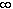#Interactive Real Analysis

Next | Previous | Glossary | Map

## 5.1. Open and Closed Sets

### Examples 5.1.2(a):

Which of the intervals (-3, 3), [4, 7], (-4, 5], (0,) and [0,) are open, closed, both, or neither ?
• The interval (-3, 3) is open, because if x is any number in (-3, 3), then -3 < x < 3. or equivalently, -3 - x < 0 < 3 - x. Now let= min( 3 + x, 3 - x ). Then> 0, and the interval (x -/ 2, x +/ 2) is contained in (-3, 3). In fact, the same argument works for any interval of the form (a, b) with a, b real numbers.
• The interval [4, 7] is closed, because its complement consists of the two open sets (-, 4) and (7,).
• The interval (-4, 5] is neither open nor closed. It is not open, because the point x = 5 is contained in the set, but every neighborhood of that point is not contained inside the set. It is not closed, because its complement (-, -4] and (5,) is not open at x = -4.
• The interval (0,) and also the interval (-, 0) are both open, because every point in the set contains a neighborhood contained inside the set.
• The interval [0,) and also the interval (-,0] are both closed, because their complements are the open sets mentioned above.
Next | Previous | Glossary | Map